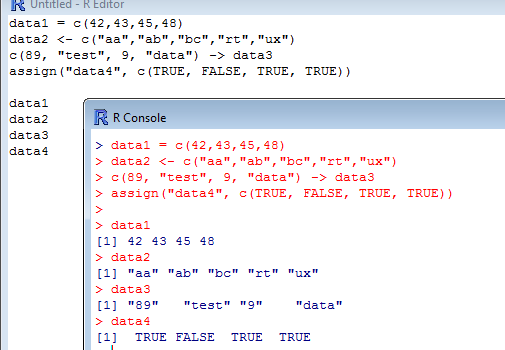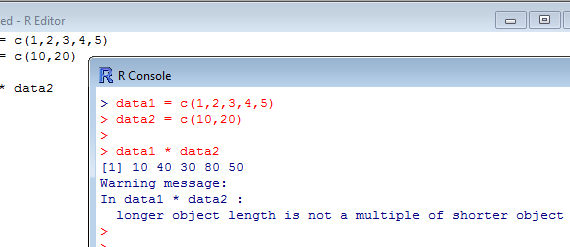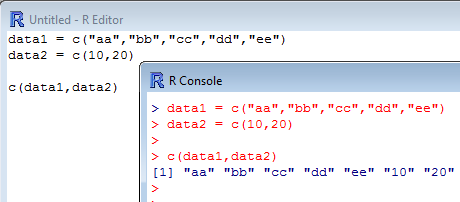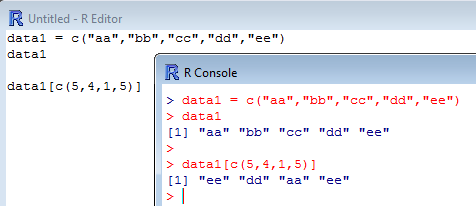R -2- Creating vectors

Vectors are simple objects used in R to stored data elements such as numbers, text or logical values (TRUE or FALSE) under a variable name. They allow you to simplify your work by saving many values under a unique name, to create plenty of such groups of data to be used, reused, combined, modified, erased (…) at your will. They allow you also to perform a multitude of simple or complex operations on a bunch of data elements in a minimal amount of steps.

1. Choosing a name for your vectorIn R, storing data under a specific name is called assignment. There are at least 4 ways to assign data in R: a. with the operator = b. with the operator <- c. with the operator -> d. with the function assign("name,..."). Here is a practical example where single values […]

2. Assigning data elements to a vectorNow that you have stored values or data elements in vectors, you may start working with/on these vectors. The simplest operations that you can perform are regular arithmetical operations such as addition, subtraction, multiplication and division. Here is an example where the vector data1 is multiplied by 5: [code language=”r”] […]

3. Simple arithmetical operations involving vectorsNot only you can perform arithmetic operations to vectors, but you can also “merge” them or combine them. To do so, simply use the concatenate function c(...) to concatenate the two vectors,: [code language=”r”] data1 = c("aa","bb","cc","dd","ee") data2 = c(10,20) c(data1,data2) [/code]   The example above is a bit particular […]

4. Combining vectorsA good thing when working with vectors is that R does not try to reorder the data elements to be stored upon assignment. This means that the order in which the elements have been concatenated remains! Thus, one can easily call any element of a vector by mentioning its position […]